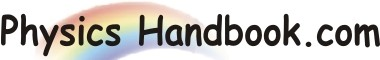HOME TOPICS DEFINITIONS TABLES LAWS INVENTIONS EXPERIMENTS QUIZ VIDEOS
 A B C D E F G H I J K L M N O P Q R S T U V W X Y Z
Combination of Basic Logic Gates
The OR, AND and NOT gates are the three basic circuits that make up all digital circuits. We shall discuss a few combinations of theses basic circuits.
(I) NAND Gate: It is combination of AND gate is connected to the input of a NOT gate as shown in Fig. (a). Clearly, the output of a NAND gate is opposite to the AND gate. This is illustrated in the truth table for the NAND gate. Note that truth table for NAND gate is developed by inverting the output of the AND gate.
The Boolean expression for NAND function is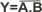This Boolean expression can be read as Y = not A . B. TO perform the Boolean algebra operation, First the input must be AND and then the inversion is performed. Note that output from NAND gate is always 1 excepted when all of the input are 1.Fig. (b) shows the logic symbol for a NAND gate. The title bubble (small circle) on the right end of the symbol means to invert the AND.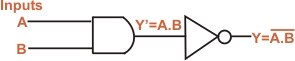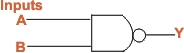Fig. (a) Fig. (b)
Inputs Outputs
A
B
AND(Y')
NAND(Y)
0
0
0
1
0
1
0
1
1
0
0
1
1
1
1
0
Truth Table of NAND
(II) NOR Gate: It is a combination of OR gate and NOT gate. In other words, output of OR gate is connected to the input of a NOT gate as shown in Fig. (c). Note that output of OR gate is inverted to form NOR gate. This is illustrated in the truth table for NOR gate. It is clear that truth table for NOR gate is developed by inverting the outputs of the OR gate.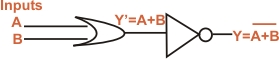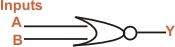Fig. (c) Fig. (d)
Inputs Outputs
A
B
OR(Y')
NOR(Y)
0
0
0
1
0
1
1
0
1
0
1
0
1
1
1
0
Truth Table of NOR
The Boolean expression for NOR function is: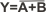The Boolean expression can be read as Y = not A or B. To perform the Boolean algebra operation, first the input must be OR and then inversion is performed. Note that output from a NOR gate is high(1) only when all the inputs are low (0). If any of the inputs are high (1) the output is low (0). fig. (d) shows the logic symbol for a NOR gate. The bubble (small circle) at the Y output indicates inversion.